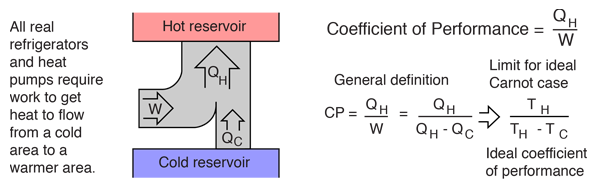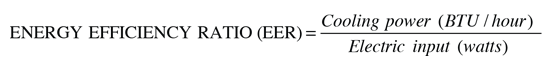# Heat Pump

A heat pump is a device which applies external work to extract an amount of heat QC from a cold reservoir and delivers heat QH to a hot reservoir. A heat pump is subject to the same limitations from the second law of thermodynamics as any other heat engine and therefore a maximum efficiency can be calculated from the Carnot cycle. Heat Pumps are usually characterized by a coefficient of performance which is the number of units of energy delivered to the hot reservoir per unit work input.Note: In calculating the coefficient of performance, or any other heat-engine related quantities, the temperatures must be the values in Kelvins.

 General comments on air conditioners and heat pumps
Index

Heat engine concepts

 HyperPhysics***** Thermodynamics R Nave
Go Back

# Air Conditioners and Heat Pumps

Air conditioners and heat pumps are heat engines like the refrigerator. They make good use of the high quality and flexibility of electric energy in that they can use one unit of electric energy to transfer more than one unit of energy from a cold area to a hot area. For example, an electric resistance heater using one kilowatt-hour of electric energy can transfer only 1 kWh of energy to heat your house at 100% efficiency. But 1 kWh of energy used in an electric heat pump could "pump" 3 kWh of energy from the cooler outside environment into your house for heating. The ratio of the energy transferred to the electric energy used in the process is called its coefficient of performance (CP). A typical CP for a commercial heat pump is between 3 and 4 units transferred per unit of electric energy supplied.

 Heat pump energy flow sketch Coefficient of performance Energy efficiency ratio
Index

PV diagram concepts

Heat engine concepts

 HyperPhysics***** Thermodynamics R Nave
Go Back

# Coefficient of Performance

The coefficient of performance (CP) for a heat pump is the ratio of the energy transferred for heating to the input electric energy used in the process. In reference to the standard heat engine illustration, the coefficient is defined byThere is a theoretical maximum CP, that of the Carnot cycle :For a refrigerator, however, the useful quantity is the heat extracted, QC , not the heat exhausted. Therefore, the coefficient of performance of a refrigerator is expressed asFor consumer refrigerators in the U.S., the coefficient of performance for refrigerators is typically recast into a number called the Energy Efficiency Ratio.

Index

PV diagram concepts

Heat engine concepts

 HyperPhysics***** Thermodynamics R Nave
Go Back

# Energy Efficiency Ratio

The efficiencies of air conditioners and heat pumps sold in the United States are often stated in terms of an energy efficiency ratio (EER):This peculiar ratio can be compared to the more straightforward coefficient of performance by converting BTU/hr to watts:Therefore CP = EER x 0.292. The range of EER's for air conditioners is typically about 5.5 to 10.5 with those units for which EER>7.5 being classified as "high efficiency" units. This is a range of 1.6 to 3.1 in CP.

Index

Heat engine concepts

 HyperPhysics***** Thermodynamics R Nave
Go Back

# Heat Pump Energy FlowThe electric heat pump can overcome the thermal bottleneck imposed by the second law of thermodynamics for the purpose of heating a house. As the illustration shows, an electric heat pump can deliver more heat to a house than burning the primary fuel at 100% efficiency inside the house. That is higher efficiency than a typical forced-air natural gas furnace which brings the primary fuel to the house. This comparison is not quite fair to the natural gas, however, since you can purchase natural gas furnaces which deliver the gas energy into heating at over 90% efficiency.

 Example of home heating costs.
Index

Heat engine concepts

 HyperPhysics***** Thermodynamics R Nave
Go Back